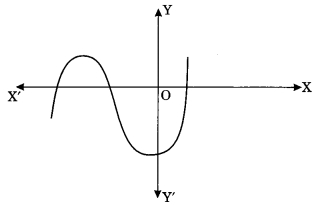# CBSEtips.in

## Thursday, 28 January 2021

### CBSE Class 10 Maths - MCQ and Online Tests - Unit 2 - Polynomials

#### CBSE Class 10 Maths – MCQ and Online Tests – Unit 2 – Polynomials

Every year CBSE conducts board exams for 10th standard. These exams are very competitive to all the students. So our website provides online tests for all the 10th subjects. These tests are also very effective and useful for those who preparing for competitive exams like NEET, JEE, CA etc. It can boost their preparation level and confidence level by attempting these chapter wise online tests.

These online tests are based on latest CBSE Class 10 syllabus. While attempting these our students can identify the weak lessons and continuously practice those lessons for attaining high marks. It also helps to revise the NCERT textbooks thoroughly.

#### CBSE Class 10 Maths – MCQ and Online Tests – Unit 2 – Polynomials

Question 1.
The maximum number of zeroes that a polynomial of degree 4 can have is
(a) One
(b) Two
(c) Three
(d) Four

Question 2.
The graph of the polynomial p(x) = 3x – 2 is a straight line which intersects the x-axis at exactly one point namely
(a) ($$\frac{-2}{3}$$, 0)
(b) (0, $$\frac{-2}{3}$$)
(c) ($$\frac{2}{3}$$, 0)
(d) $$\frac{2}{3}$$, $$\frac{-2}{3}$$

Answer: (c) ($$\frac{2}{3}$$, 0)

Question 3.
In fig. given below, the number of zeroes of the polynomial f(x) is(a) 1
(b) 2
(c) 3
(d) None

Question 4.
The graph of the polynomial ax² + bx + c is an upward parabola if
(a) a > 0
(b) a < 0
(b) a = 0
(d) None

Question 5.
The graph of the polynomial ax² + bx + c is a downward parabola if
(a) a > 0
(b) a < 0
(c) a = 0
(d) a = 1

Question 6.
A polynomial of degree 3 is called
(a) a linear polynomial
(c) a cubic polynomial

Question 7.
If α, β are the zeroes of the polynomial x² – 16, then αβ(α + β) is
(a) 0
(b) 4
(c) -4
(d) 16

Question 8.
If α and $$\frac{1}{α}$$ are the zeroes of the polynomial ax² + bx + c, then value of c is
(a) 0
(b) a
(c) -a
(d) 1

Question 9.
Zeroes of the polynomial x² – 11 are
(a) ±$$\sqrt{17}$$
(b) ±$$\sqrt{3}$$
(c) 0
(d) None

Answer: (a) ±$$\sqrt{17}$$

Question 10.
If α, β, γ are the zeroes of the cubic polynomial ax³ + bx² + cx + d then α + β + γ is equal
(a) $$\frac{-b}{a}$$
(b) $$\frac{b}{a}$$
(c) $$\frac{c}{a}$$
(d) $$\frac{d}{a}$$

Answer: (a) $$\frac{-b}{a}$$

Question 11.
If α, β, γ are the zeroes of the cubic polynomial ax³ + bx² + cx + d then αβ + βγ + αγ is equal to
(a) $$\frac{-b}{a}$$
(b) $$\frac{b}{a}$$
(c) $$\frac{c}{a}$$
(d) $$\frac{d}{a}$$

Answer: (c) $$\frac{c}{a}$$

Question 12.
If the zeroes of the polynomial x³ – 3x² + x – 1 are $$\frac{s}{t}$$, s and st then value of s is
(a) 1
(b) -1
(c) 2
(d) -3

Question 13.
If the sum of the zeroes of the polynomial f(x) = 2x³ – 3kx² + 4x – 5 is 6, then the value of k is
(a) 2
(b) 4
(c) -2
(d) -4

Question 14.
If a polynomial of degree 4 is divided by quadratic polynomial, the degree of the remainder is
(a) ≤ 1
(b) ≥ 1
(c) 2
(d) 4

Question 15.
If a – b, a and a + b are zeroes of the polynomial fix) = 2x³ – 6x² + 5x – 7, then value of a is
(a) 1
(b) 2
(c) -5
(d) 7

Question 16.
Dividend is equal to
(a) divisor × quotient + remainder
(b) divisior × quotient
(c) divisior × quotient – remainder
(d) divisor × quotient × remainder

Answer: (a) divisor × quotient + remainder

Question 17.
A quadratic polynomial whose sum of the zeroes is 2 and product is 1 is given by
(a) x² – 2x + 1
(b) x² + 2x + 1
(c) x² + 2x – 1
(d) x² – 2x – 1

Answer: (a) x² – 2x + 1

Question 18.
If one of the zeroes of a quadratic polynomial ax² + bx + c is 0, then the other zero is
(a) $$\frac{-b}{a}$$
(b) 0
(c) $$\frac{b}{a}$$
(d) $$\frac{-c}{a}$$

Answer: (a) $$\frac{-b}{a}$$

Question 19.
The sum and the product of the zeroes of polynomial 6x² – 5 respectively are
(a) 0, $$\frac{-6}{5}$$
(b) 0, $$\frac{6}{5}$$
(c) 0, $$\frac{5}{6}$$
(d) 0, $$\frac{-5}{6}$$

Answer: (d) 0, $$\frac{-5}{6}$$

Question 20.
What should be subtracted from x³ – 2x² + 4x + 1 to get 1?
(a) x³ – 2x² + 4x
(b) x³ – 2x² + 4 + 1
(c) -1
(d) 1

Answer: (a) x³ – 2x² + 4x

Share: